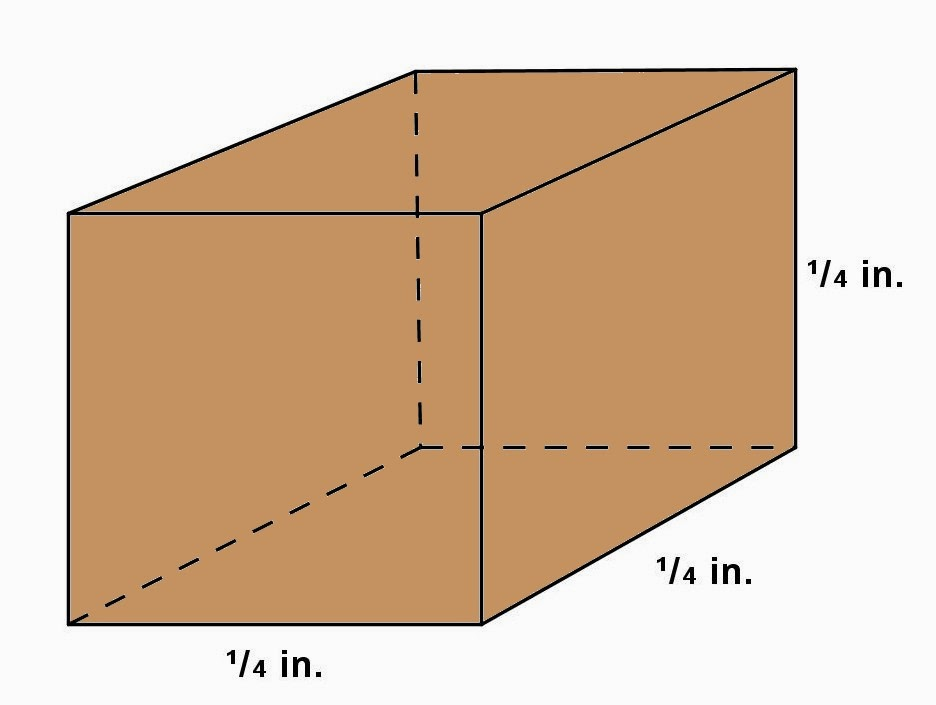## Wednesday, April 30, 2014

### More Cube Problems, 3

Category: Solid Geometry, Physics

"Published in Newark, California, USA"

What is the weight of a block of ice 24 in. by 24 in. by 24 in., if ice weighs 92 percent as much as water, and water weighs 62.5 lb. per cu. ft.?

Solution:

To illustrate the problem, it is better to draw the figure as followsPhoto by Math Principles in Everyday Life

The first that we need to do is to get the volume of an ice which is a cube as follows

Since the density of ice is expressed in lb. per cu.ft., then we need to convert the volume of an ice in cu. ft. as follows

If the specific gravity of ice is 0.92, then the density of ice is

Therefore, the weight of ice is

## Tuesday, April 29, 2014

### More Cube Problems, 2

Category: Solid Geometry

"Published in Newark, California, USA"

Plato (429 - 348 B.C.) was one of the first to discover a solution to that famous problem of antiquity, the duplication of a cube, i.e., the finding of the edge of a cube whose volume is double that of a given cube.

One legend asserts that the Athenians, who were suffering from a plague of typhoid fever, consulted the oracle at Delos as to how to stop the plague. Apollo replied that the Delians would have to double the size of his altar, which was in the form of a cube. A new altar was constructed having its edge twice as long as that of the old one. But the pestilence became worse than before, whereupon the Delians appealed to Plato. Given that the side of the altar was 8 ft., find, accurate to five figures, the edge of the required altar.

Solution:

To illustrate the problem, it is better to draw the figure as followsPhoto by Math Principles in Everyday Life

Consider the first cube or old altar:

Consider the second cube or new altar:

Therefore, the edge of the required or new altar is

## Monday, April 28, 2014

### More Cube Problems

Category: Solid Geometry

"Published in Vacaville, California, USA"

How much material was used in the manufacture of 24,000 celluloid dice, if each die has an edge of ¼ in.?

Solution:

To illustrate the problem, it is better to draw the figure as followsPhoto by Math Principles in Everyday Life

The volume of a cube is

Therefore, the amount of material used in making 24,000 celluloid dice is

## Sunday, April 27, 2014

### More Triangle Problems, 7

Category: Plane Geometry

"Published in Vacaville, California, USA"

A pole 26 ft. long leans against a wall at a point 10 ft. from the ground. What is the length of the projection of the pole on the ground?

Solution:

To illustrate the problem, it is better to draw the figure as followsPhoto by Math Principles in Everyday Life

Let's assume that the wall and the ground are perpendicular to each other.

Let y = be the length of the projection of the pole on the ground.

By Pythagorean Theorem, the length of the projection of the pole on the ground is

## Saturday, April 26, 2014

### Similar Triangles, 2

Category: Plane Geometry

"Published in Vacaville, California, USA"

A street light is 15 ft. directly above the curb. A man 6 ft. tall starts 10 ft. down street from the light and walks directly across the street which is 10 ft. wide. When he reaches the opposite curb, what is the distance between the initial and final positions of the tip of his shadow?

Solution:

To illustrate the problem, it is better to draw the figure as followsPhoto by Math Principles in Everyday Life

Let x = be the length of his shadow at the 1st curb.

By using similar triangles

If a man walks 10 ft. directly across the street which is at the opposite curb, the figure above becomesPhoto by Math Principles in Everyday Life

In order to solve for the distance between the initial and final positions of the tip of his shadow, let's label further the above figure as followsPhoto by Math Principles in Everyday Life

Let a = be the distance of a man to the street light from 2nd curb
b = be the length of his shadow at the 2nd curb
y = be the distance between the initial and final positions of the tip of his shadow

By Pythagorean Theorem

By using similar triangles

Since the 1st Curb is parallel to the 2nd Curb, then the small and large triangles are similar to each other. If the small triangle is an isosceles right triangle, then the large triangle is also an isosceles right triangle.

By Pythagorean Theorem

which is the same as the length of a man's shadow at the 1st Curb.

Therefore, the distance between the initial and final positions of the tip of his shadow is